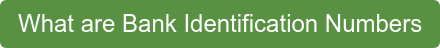# What is a Luhn Check?

Luhn checks are algorithmic checks used to approve credit cards. These checks used the Luhn algorithm to determine single-digit mistakes made when entering digits. This is mostly used for credit card authentication to identify when cardholders mistype numbers. The Luhn algorithm is able to reject invalid card numbers, whether they are entered through human error or clumsy malicious attacks.

Luhn checks are created to identify inaccurately entered card details, not mitigate fraud. The Luhn algorithm will identify invalid cards whose digits have been swapped or inaccurately entered as typos. However, Luhn checks can counter hackers who don’t take the time to understand the makeup of card numbers. More experienced fraudsters, on the other hand, will be able to use the Luhn formula which exists in the public domain to generate potential card numbers.

# What is the Luhn Algorithm?

The mod 10 algorithm, also known as the Luhn algorithm, is the algorithm used to complete a Luhn check. The Luhn algorithm checks whether a card number is input correctly by checking both the number and value of digits entered. The final number is used as a check to ensure that the card number is valid. This simple calculation helps separate potentially valid numbers from randomly generated numbers.

# How does the Luhn Algorithm Calculation work?

The Luhn Algorithm uses a simple calculation to determine whether a transaction is valid. Here is the calculation:

• Every other digit (moving right to left, starting with the second to last digit) is doubled and added together. Additionally, if doubling a digit creates a two digit number (like 12) the digits are added together (1+2=3)
• The skipped over digits are added together
• The resulting values from the two groups are added together for the final sum
• If the resulting value ends in a 0, the card number is valid
•

Let’s look at an example of how the calculation would work in practice with an example Visa number:

• A customer enters the card number 4147 2030 5978 0942
• Every other digit is doubled:

4x2= 8

0x2= 0

7x2= 14

5x2= 10

3x2= 6

2x2 = 4

4x2= 8

4x2=8

• The digits generated are added together:

8+0+1+4+1+0+6+4+8+8 = 40

• The remaining digits are also added together:

2+9+8+9+0+0+7+1 = 36

• The two groups are added together:

40 + 36 = 76

• 76 does not end in 0, which means this card number is not valid

We can see that this card number has failed the Luhn check. However, the change of a single digit would make the card number valid. If the number 3 is changed to a 0 (the invalid card number 4147 2030 5978 0942 is changed to 4147 2000 5978 0942) and the calculation is rerun, it will pass the Luhn check.

It should be noted that some people separate the first 15 digits that are entered into the Luhn algorithm, while reserving the last digit as the check digit. Mathematically, adding the last digit after everything else doesn’t change the final sum. As long as the final sum ends with 0 (ie. is divisible by 10), the card number passes the Luhn check.

Luhn checks are incredibly valuable for identifying invalid card information, especially mistakenly entered card information. The Luhn algorithm is just one of the many factors that goes into creating a card number. Between BINs (Bank Identification Numbers), CVVs, and the Luhn algorithm, there are many different rules that work together to make card information hard to hack.

If you’re interested in the building blocks of credit card numbers, click below to read our blog on BINs and what they mean for the safety and storage of cardholder information.Topic(s): payments